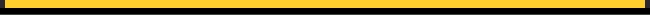Quarterback Ratings: How Does it Work? Source: 2004 National Football League Record & Fact Book  The QB Rating has become the standard benchmark for gauging the success of QBs, despite the era in which they played or what kind of offenses they ran. I've always been curious how the rating is determined, especially since it always seemed weird that a perfect rating is 158.33. Why isn't is a base-ten system where 100 is the perfect score? A visit to the NFL.com history section provided the following answers:   The NFL rates its passers for statistical purposes against a fixed performance standard based on statistical achievements of all qualified pro passers since 1960. The current system replaced one that rated passers in relation to their position in a total group based on various criteria. The current system, which was adopted in 1973, removes inequities that existed in the former method and, at the same time, provides a means of comparing passing performances from one season to the next. It is important to remember that the system is used to rate passers, not quarterbacks. Statistics do not reflect leadership, play-calling, and other intangible factors that go into making a successful professional quarterback. Four categories are used as a basis for compiling a rating: Percentage of completions per attempt Average yards gained per attempt Percentage of touchdown passes per attempt Percentage of interceptions per attempt The average standard is 1.000. The bottom is .000. To earn a 2.000 rating, a passer must perform at exceptional levels, i.e., 70 percent in completions, 10 percent in touchdowns, 1.5 percent in interceptions, and 11 yards average gain per pass attempt. The maximum a passer can receive in any category is 2.375. For example, to gain a 2.375 in completion percentage, a passer would have to complete 77.5 percent of his passes. The NFL record is 70.55 by Ken Anderson (Cincinnati, 1982). To earn a 2.375 in percentage of touchdowns, a passer would have to achieve a percentage of 11.9. The record is 13.9 by Sid Luckman (Chicago, 1943). To gain 2.375 in percentage of interceptions, a passer would have to go the entire season without an interception. The 2.375 figure in average yards is 12.50, compared with the NFL record of 11.17 by Tommy O'Connell (Cleveland, 1957). In order to make the rating more understandable, the point rating is then converted into a scale of 100, with 158.3 being the highest rating a passer can achieve. In cases where statistical performance has been superior, it is possible for a passer to surpass a 100 rating. For example, take Steve Young's record-setting season in 1994 when he completed 324 of 461 passes for 3,969 yards, 35 touchdowns, and 10 interceptions. The four calculations would be: Percentage of Completions 324 of 461 is 70.28 percent. Subtract 30 from the completion percentage (40.28) and multiply the result by 0.05. The result is a point rating of 2.014. Note: If the result is less than zero (Comp. Pct. less than 30.0), award zero points. If the results are greater than 2.375 (Comp. Pct. greater than 77.5), award 2.375. Average Yards Gained Per Attempt 3,969 yards divided by 461 attempts is 8.61. Subtract three yards from yards-per-attempt (5.61) and multiply the result by 0.25. The result is 1.403. Note: If the result is less than zero (yards per attempt less than 3.0), award zero points. If the result is greater than 2.375 (yards per attempt greater than 12.5), award 2.375 points. Percentage of Touchdown Passes 35 touchdowns in 461 attempts is 7.59 percent. Multiply the touchdown percentage by 0.2. The result is 1.518. Note: If the result is greater than 2.375 (touchdown percentage greater than 11.875), award 2.375. Percentage of Interceptions 10 interceptions in 461 attempts is 2.17 percent. Multiply the interception percentage by 0.25 (0.542) and subtract the number from 2.375. The result is 1.833. Note: If the result is less than zero (interception percentage greater than 9.5), award zero points. The sum of the four steps is (2.014 + 1.403 + 1.518 + 1.833) 6.768. The sum is then divided by six (1.128) and multiplied by 100. In this case, the result is 112.8. This same formula can be used to determine a passer rating for any player who attempts at least one pass.